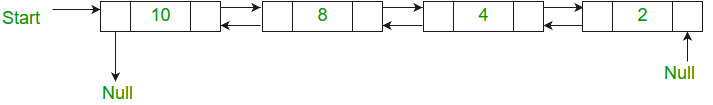GFG App
Open AppBrowser
Continue

# Java Program For Merge Sort For Doubly Linked List

Given a doubly linked list, write a function to sort the doubly linked list in increasing order using merge sort.
For example, the following doubly linked list should be changed to 24810Merge sort for singly linked list is already discussed. The important change here is to modify the previous pointers also when merging two lists.

Below is the implementation of merge sort for doubly linked list.

## Java

 `// Java program to implement merge sort in  ` `// singly linked list ` `// Linked List Class ` `class` `LinkedList  ` `{ ` `    ``// Head of list ` `    ``static` `Node head;   ` ` `  `    ``// Node Class  ` `    ``static` `class` `Node  ` `    ``{ ` `        ``int` `data; ` `        ``Node next, prev; ` ` `  `        ``// Constructor to create a  ` `        ``// new node ` `        ``Node(``int` `d)  ` `        ``{ ` `            ``data = d; ` `            ``next = prev = ``null``; ` `        ``} ` `    ``} ` ` `  `    ``void` `print(Node node)  ` `    ``{ ` `        ``Node temp = node; ` `        ``System.out.println( ` `        ``"Forward Traversal using next pointer"``); ` `        ``while` `(node != ``null``)  ` `        ``{ ` `            ``System.out.print(node.data + ``" "``); ` `            ``temp = node; ` `            ``node = node.next; ` `        ``} ` `        ``System.out.println( ` `        ``"Backward Traversal using prev pointer"``); ` `        ``while` `(temp != ``null``)  ` `        ``{ ` `            ``System.out.print(temp.data + ``" "``); ` `            ``temp = temp.prev; ` `        ``} ` `    ``} ` ` `  `    ``// Split a doubly linked list (DLL) into  ` `    ``// 2 DLLs of half sizes ` `    ``Node split(Node head)  ` `    ``{ ` `        ``Node fast = head, slow = head; ` `        ``while` `(fast.next != ``null` `&&  ` `               ``fast.next.next != ``null``)  ` `        ``{ ` `            ``fast = fast.next.next; ` `            ``slow = slow.next; ` `        ``} ` `        ``Node temp = slow.next; ` `        ``slow.next = ``null``; ` `        ``return` `temp; ` `    ``} ` ` `  `    ``Node mergeSort(Node node)  ` `    ``{ ` `        ``if` `(node == ``null` `||  ` `            ``node.next == ``null``)  ` `        ``{ ` `            ``return` `node; ` `        ``} ` `        ``Node second = split(node); ` ` `  `        ``// Recur for left and right halves ` `        ``node = mergeSort(node); ` `        ``second = mergeSort(second); ` ` `  `        ``// Merge the two sorted halves ` `        ``return` `merge(node, second); ` `    ``} ` ` `  `    ``// Function to merge two linked lists ` `    ``Node merge(Node first, Node second)  ` `    ``{ ` `        ``// If first linked list is empty ` `        ``if` `(first == ``null``)  ` `        ``{ ` `            ``return` `second; ` `        ``} ` ` `  `        ``// If second linked list is empty ` `        ``if` `(second == ``null``)  ` `        ``{ ` `            ``return` `first; ` `        ``} ` ` `  `        ``// Pick the smaller value ` `        ``if` `(first.data < second.data)  ` `        ``{ ` `            ``first.next = merge(first.next,  ` `                               ``second); ` `            ``first.next.prev = first; ` `            ``first.prev = ``null``; ` `            ``return` `first; ` `        ``}  ` `        ``else`  `        ``{ ` `            ``second.next = merge(first,  ` `                                ``second.next); ` `            ``second.next.prev = second; ` `            ``second.prev = ``null``; ` `            ``return` `second; ` `        ``} ` `    ``} ` ` `  `    ``// Driver code ` `    ``public` `static` `void` `main(String[] args)  ` `    ``{ ` `        ``LinkedList list = ``new` `LinkedList(); ` `        ``list.head = ``new` `Node(``10``); ` `        ``list.head.next = ``new` `Node(``30``); ` `        ``list.head.next.next = ``new` `Node(``3``); ` `        ``list.head.next.next.next =  ` `        ``new` `Node(``4``); ` `        ``list.head.next.next.next.next =  ` `        ``new` `Node(``20``); ` `        ``list.head.next.next.next.next.next =  ` `        ``new` `Node(``5``);         ` `         `  `        ``Node node = ``null``; ` `        ``node = list.mergeSort(head); ` `        ``System.out.println( ` `        ``"Linked list after sorting :"``); ` `        ``list.print(node); ` `    ``} ` `} ` `// This code is contributed by Mayank Jaiswal `

Output:

```Linked List after sorting
Forward Traversal using next pointer
3 4 5 10 20 30
Backward Traversal using prev pointer
30 20 10 5 4 3```

Time Complexity: Time complexity of the above implementation is same as time complexity of MergeSort for arrays. It takes Θ(nLogn) time.

Space Complexity:O(1). We are only using constant amount of extra space.
You may also like to see QuickSort for doubly linked list
Please refer complete article on Merge Sort for Doubly Linked List for more details!

My Personal Notes arrow_drop_up